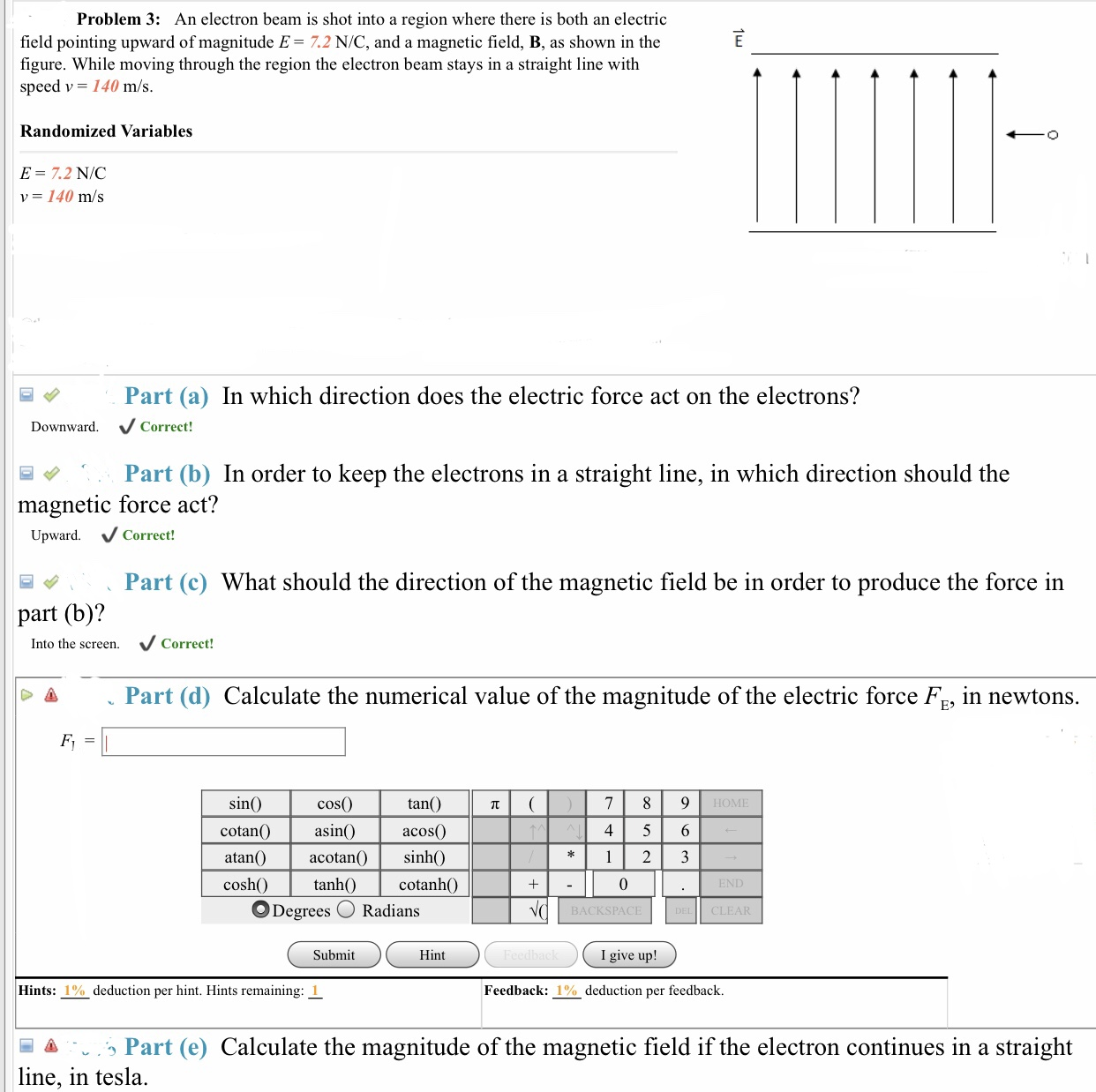# Problem 3: An electron beam is shot into a region where there is both an electric field pointing upward of magnitude E- 7.2 N/C, and a magnetic field, B, as shown in the figure. While moving through the region the electron beam stays in a straight line with speed v- 140 m/s Randomized Variables E = 7.2 N/C v= 140 m/s Part (a) In which direction does the electric force act on the electrons? Downward. Correct! Part (b) In order to keep the electrons in a straight line, in which direction should the magnetic force act? ward. Correct! Part (c) What should the direction of the magnetic field be in order to produce the force in part (b)? Into the screen. Correct! Part (d) Calculate the numerical value of the magnitude of the electric force Fe, in newtons. cos(0) asin() atan() acotan sinh() sin tan() HOME cotanO a acos 4 5 6 cosh cotanhO O Degrees Radians BACKSPACE CLEAR Submit Hint I give up! Hints: 1% deduction per hint. Hints remaining: 1 Feedback: 1%deduction per feedback. .., Part (e) Calculate the magnitude of the magnetic field if the electron continues in a straight line, in tesla.

Questionhelp_outlineImage TranscriptioncloseProblem 3: An electron beam is shot into a region where there is both an electric field pointing upward of magnitude E- 7.2 N/C, and a magnetic field, B, as shown in the figure. While moving through the region the electron beam stays in a straight line with speed v- 140 m/s Randomized Variables E = 7.2 N/C v= 140 m/s Part (a) In which direction does the electric force act on the electrons? Downward. Correct! Part (b) In order to keep the electrons in a straight line, in which direction should the magnetic force act? ward. Correct! Part (c) What should the direction of the magnetic field be in order to produce the force in part (b)? Into the screen. Correct! Part (d) Calculate the numerical value of the magnitude of the electric force Fe, in newtons. cos(0) asin() atan() acotan sinh() sin tan() HOME cotanO a acos 4 5 6 cosh cotanhO O Degrees Radians BACKSPACE CLEAR Submit Hint I give up! Hints: 1% deduction per hint. Hints remaining: 1 Feedback: 1%deduction per feedback. .., Part (e) Calculate the magnitude of the magnetic field if the electron continues in a straight line, in tesla. fullscreen

### Want to see the step-by-step answer?